### Home > CC3 > Chapter Ch10 > Lesson 10.1.2 > Problem10-29

10-29.
1. Simplify each expression. Homework Help ✎

1.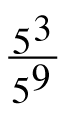2.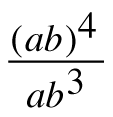3. 8x–3(8x)2

4.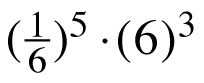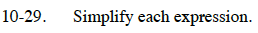What do the exponents mean?

$\frac{5\cdot 5\cdot 5}{5\cdot 5\cdot 5\cdot 5\cdot 5\cdot 5\cdot 5\cdot 5\cdot 5}$

Simplify.

$\frac{1}{5^6}=5^{-6}\footnotesize$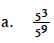See part (a).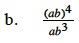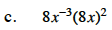What do the exponents mean?
Be mindful of the parentheses.

$\frac{8\left(8\it\; x\right)^2}{\it\; x^3}$

Expand the exponents.

$\frac{8\cdot 8\cdot 8\cdot \it\; x\cdot \it\; x}{x\cdot x\cdot x}$

$\frac{8^3}{\it x}$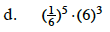See part (c).